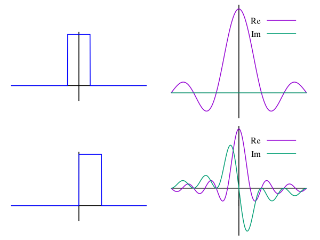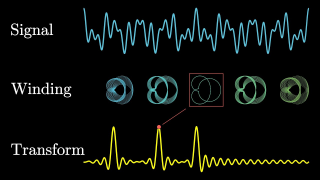# Fourier transform

Topic | v1 | created by janarez |Description

In mathematics, a Fourier transform (FT) is a mathematical transform that decomposes functions depending on space or time into functions depending on spatial or temporal frequency, such as the expression of a musical chord in terms of the volumes and frequencies of its constituent notes. The term Fourier transform refers to both the frequency domain representation and the mathematical operation that associates the frequency domain representation to a function of space or time. The Fourier transform of a function of time is a complex-valued function of frequency, whose magnitude (absolute value) represents the amount of that frequency present in the original function, and whose argument is the phase offset of the basic sinusoid in that frequency. The Fourier transform is not limited to functions of time, but the domain of the original function is commonly referred to as the time domain.

##### Resources

10.0 rating 2.0 level 10.0 clarity 4.0 background – 1 rating

An animated introduction to the Fourier Transform.The Fourier Transform is one of deepest insights ever made. Unfortunately, the meaning is buried with...